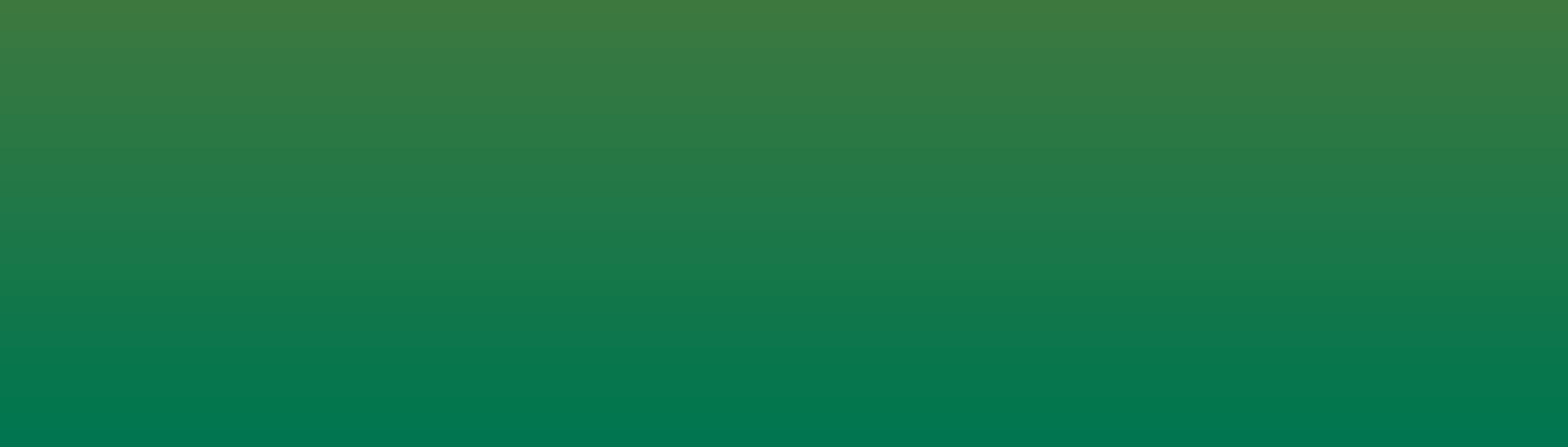See on GitHub# Install

git clone https://github.com/JakeRoggenbuck/colorgradient-rs


# Test

cargo test


# Code

fn calculate_gradient(num: i64, original_colors: Vec<RGB>) -> Vec<RGB> {
// Get the needed step value to fit the num of iterations in the original_colors length
let step: f32 = (original_colors.len() as f32 - 1.0) / num as f32;
let channels = get_channels(original_colors);
let mut colors = Vec::<RGB>::new();

for i in 0..num {
// Use step to count up with index
let x: f32 = i as f32 * step;
let mut color = Vec::new();
// Get each channel as a reference, use it as the known x values
for channel in [&channels.red, &channels.green, &channels.blue].iter() {
// Add the y values found from the x value to the color
color.push(find_y(x as f32, channel).abs());
}
// Change the vector of colors to RGB structure, and add it to all the colors
colors.push(vec_to_rgb!(color));
}
return colors;
}